Courses

Test: Electricity - 2

20 Questions MCQ Test Science & Technology for UPSC CSE | Test: Electricity - 2

Description
Attempt Test: Electricity - 2 | 20 questions in 20 minutes | Mock test for Class 10 preparation | Free important questions MCQ to study Science & Technology for UPSC CSE for Class 10 Exam | Download free PDF with solutions
QUESTION: 1

The correct relation between current 'I' and amount of charge flown 'q' during a time 't' through a conductor is

Solution:

Correct relation is current I =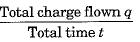⇒ q = It

QUESTION: 2

The effective resistance between A and B is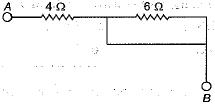Solution:

6Ω is shorted so effective resistance is 4Ω.

QUESTION: 3

SI unit of resistivity is

Solution:

As resistivity of the material of a resistor of length l, cross-section area A and resistance R is given by :ρ = R.A/I, hence SI unit of resistivity is ohm -metre (Ω m).

QUESTION: 4

A cooler of 1500 W, 200 volt and a fan of 500 W, 200 volt are to be used from a household supply. The rating of fuse to be used is

Solution:

Total power used, P - P1 + P2 = 1500 + 500 = 2000 W.
Current drawn from the supply,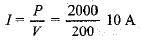QUESTION: 5

If four resistors each of 1Ω are connected in parallel, the effective resistance will be

Solution:

If four resistors each of 1Ω are connected in parallel, the effective resistance will be 0.25Ω..

As, in parralel connection,

1/Reff. = 1/R1 + 1/R2 + ....

QUESTION: 6

A fuse wire repeatedly gets burnt when used with a good heater. It is advised to use a fuse wire of

Solution:

In order to get the working of heater properly, fused wire of higher rating must be used.

QUESTION: 7

Resistivity of a given conductor depends on

Solution:

Resistivity of a given conductor depends only on its material and the temperature. Resistivity does not depend on the dimensions of the conductor.

QUESTION: 8

A coil in the heater consume power P on passing current. If it is cut into halves and joined in parallel, it will consume power

Solution:

Original power consumed, P = V2/R
When used in parallel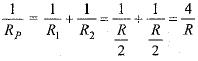RP = R/4
∴ New power consumed when two halves in parallel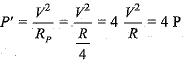QUESTION: 9

A combination of three resistances has been shown here. Effective resistance between the points A and B is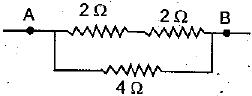Solution:

Value of series combination of 2Ω and 2Ω resistances = 4Ω. As it is now connected in parallel to 4Ω resistance, hence the effective resistance R between the points A and B will be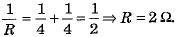QUESTION: 10

If P and V are the power and potential of device, the power consumed with a supply potential Vis

Solution: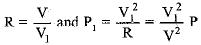QUESTION: 11

Which alloy is used to prepare the heating element of an electric iron ?

Solution:

An alloy ‘nichrome’ is used to prepare the heating element of an electric iron because its resistivity is high, its melting point is high and it does not oxidise easily.

QUESTION: 12

The resistance of hot filament of the bulb is about 10 times the cold resistance. What will be the resistance of 100 W-220 V lamp, when not in use ?

Solution:

When the lamp is on use, it is hot.
Using joules law of heating ;
P =  V2 / R
R  =  2202 /100  = 484 ohm.
It is given resistance of hot lamp is 20 times the cold lamp.
Hence, R cold  = 484/10 = 48.4 ohm.

QUESTION: 13

Two electric lamps are rated as 220 V, 100 W and 220 V, 40 W respectively. Their electric resistances are

Solution:

Resistance R = V2/P where V = voltage/potential difference and P - power
∴ electrical resistance of 100 W lamp,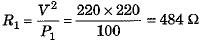and electrical resistance of 40 W lamp,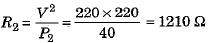QUESTION: 14

If R1 and R2 be the resistance of the filament of 40 W and 60 W respectively operating 220 V, then

Solution:

Using power,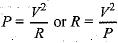For the same voltage, R ∝ 1/P
More the power, lesser the resistance.
Accordingly, R2 < R1

QUESTION: 15

A wire of resistance 8Ω is bent in the form of a closed circle. What is the effective resistance between the two points A and B, at the ends of any diameter of the circle ?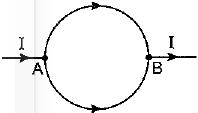Solution:

When a wire of 8Ω resistance is bent in the form of a closed circle, resistance of each half (semicircular) part is 8/2 Ω = 4Ω.
As these two parts are connected in parallel across points A and B, hence effective resistance will be 2Ω.

QUESTION: 16

Two resistors are connected in series gives an equivalent resistance of 10Ω. When connected in parallel, gives 2.4Ω. Then the individual resistance are

Solution:

In series, Rs = R1 + R2 = 1OΩ
In parallel,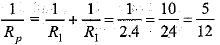QUESTION: 17

The rheostat is used in the circuit to :

Solution:

A rheostat is used to increase or decrease the magnitude of the current flowing through a circuit without change in the voltage source.

QUESTION: 18

Calculate the current flows through the 10Ω resistor in the following circuit.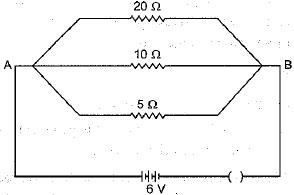Solution:

In parallel, potential difference across each resistor will remain same. So, current through 10Ω resistor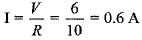QUESTION: 19

The graph between current I and potential difference V in the experimental verification of Ohm’s law were drawn by four students. Which one is correct ?

Solution:

I-V graph shown in figure (b) is correct because I ∝ 7.

QUESTION: 20

Two Wires of same length and area, made of two materials of resistivity ρ1 and ρ2 are connected in parallel V to a source of potential. The equivalent resistivity for the same length and area is

Solution: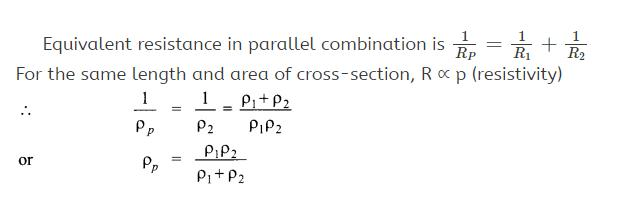Use Code STAYHOME200 and get INR 200 additional OFF Use Coupon Code

Track your progress, build streaks, highlight & save important lessons and more!

Similar ContentRelated tests